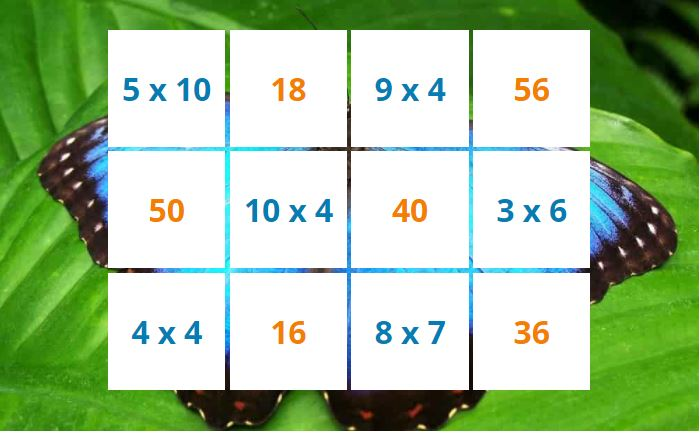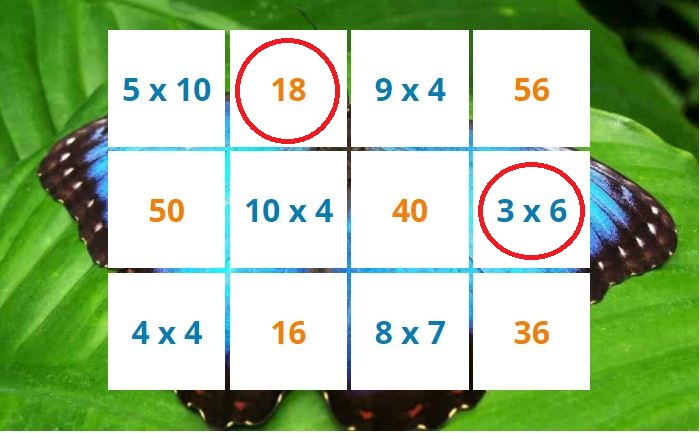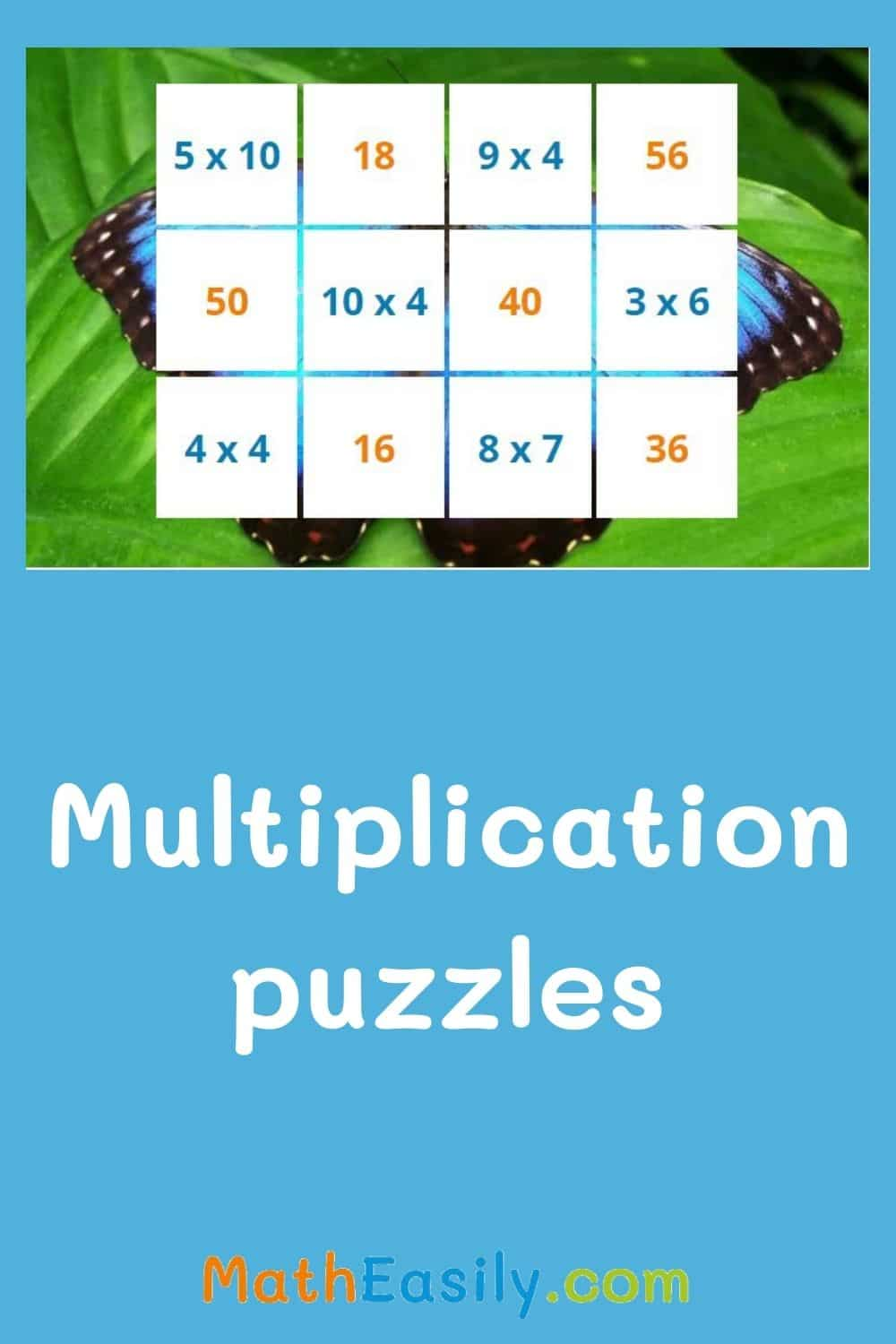﻿ Math multiplication puzzles with answers

Page No. 1064

# Multiplication puzzles

Math multiplication puzzles with answers. Play multiplication square puzzles online. multiplication picture puzzles. puzzle pics multiplication. times table puzzle. Free multiplication puzzles for grade 3. multiplication table puzzle. multiplication logic puzzles. multiplication puzzles for grade 4. Maths multiplication puzzles for grade 2. multiplication math puzzles. multiplication number puzzles. multiplication table puzzles.

Click on 2 tiles so that they contain the multiplication problem and the corresponding result.
Watch as the picture appears.

## Math multiplication puzzles with answersMultiplication picture puzzles takes advantage of children's curiosity. As kids complete the multiplication tasks, they uncover nice pictures. They look forward to what they see in the picture and therefore find the multiplication problems easy.

## Printable multiplication puzzle worksheets

These online multiplication puzzles complement well with following printable multiplication puzzle worksheets. Printable multiplication square puzzles are designed for an use in the classroom or at home. Everytime 12 new different multiplication puzzles are generated and create a new image.

Click the picture bellow and download the free multiplication puzzles in PDF:

### Multiplication picture puzzles

50 multiplication puzzles

Fun printable puzzles to practice multiplication. 50 different pictures, many multiplication tasks.

US\$ 3.00

### Multiplication math puzzles: instructions

Are you looking for a fun multiplication activity? If you are working with 2nd grade and 3rd graders, you will love these multiplication math puzzles! Children will solve simple multiplication problems to complete the multiplication puzzle game.

Students have to find out the answer for each square, then find the matching square and glue it onto it. Continue until reveal the funny picture. The best part is that this multiplication puzzle is self-correcting. Kids realise if their answers are incorrect.

• Print in color or black and white
• Cut the squares out
• Prepare some glue and scissors for each kid

### Multiplication puzzle worksheets PDF

You can also download printable multiplication puzzles in PDF below. These free multiplication puzzles with answers are generated automatically.

## Puzzle pics multiplication

Math multiplication puzzles are a great method how children learn multiplication in a fun way.

### How to play multiplication puzzles online?

Half of the tiles contain the multiplication problem (blue text) and the other half of tiles contain the result (orange text).

Click first on the multiplication problem and then on the relevant result. Looking for pairs of math multiplication puzzles: the task and the result.

When the children manage to find a pair of tiles that belong together, the tiles disappear and the hidden picture of animal appears. When kids click on 2 tiles which don´t match, the tiles will flash red.

Did you click on a tile by mistake? It doesn't matter, click it again, the tile will be white again.

Continue to play times table puzzles until all tiles disappear. Then press the button "New puzzle" and a new free multiplication puzzle will be created.## Free multiplication picture puzzles

To play multiplication square puzzles online kids need to have a basic knowledge of one digit multiplication. To practice multiplication you can play multiplication dice game online or try these multiplication flash cards.

If you prefer printed games, download this multiplication board game in PDF.

Do you like multiplication picture puzzles?
Then try also our division puzzles.## Multiplication square puzzles online

Learn multiplication easily with our multiplication puzzles for grade 3.

Usually, children solve the easiest multiplication tasks first, and at the end there remain problems they don't know so well. In this way they naturally learn multiplication.

The same principle is used for these division puzzles.